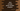# Java program to check if a number is circular prime or not## Java program to check if a number is circular prime or not:

This post will show you how to check if a number is a circular prime or not. A number is called a circular prime if it generates a prime number on all cyclic shifts of its digits. The Java program will take one number as input from the user and it will print if it is a circular prime number or not.

### Example of circular prime number:

Before we start to write the program, let me show you an example of a circular prime number.

131 is a circular prime number. Because:

• If we shift the rightmost digit of 131 to start, it will be 113
• If we shift the rightmost digit of 113 to start, it will be 311

All of these numbers, 131, 113 and 311 are prime numbers. Hence 131 is a circular prime number.

### Algorithm to check if a number is a circular prime number:

To check if a number is a circular prime number or not, we will have to:

• Take the number as input from the user.
• Find all the possible combinations of the given number.
• Calculate the total digits of the number.
• Use a loop to find all the cyclic shift combinations. The loop will run for the total digits number of times. On each iteration, pick the rightmost digit and add it to the left of the number.
• Add all numbers to a list.
• Use a loop to check for all combinations of the number. Check if all combinations are prime or not. If it fails for any combination, return False. Else, return True if all numbers are prime.
• A number is called a prime number if it has only 2 factors, 1 and the number itself.
• To check if a number is prime or not, we can run one loop that will run from 2 to number/2. On each iteration, it will check if that value can divide the number or not. If yes, it is not a prime number. Else, it is a prime number.

### Java program to check if a number is circular prime or not:

Below is the complete Java program that checks if a number is circular prime or not.

``````import java.util.ArrayList;
import java.util.Scanner;

class Main {

private static ArrayList<Integer> getCircularCombinations(int num) {
int totalDigit = String.valueOf(num).length();
int divisor = (int) (Math.pow(10, totalDigit - 1));

ArrayList<Integer> result = new ArrayList<>();

for (int i = 1; i < totalDigit; i++) {
int x = num / divisor;
int y = num % divisor;
num = y * 10 + x;
}
return result;
}

private static boolean isPrime(int num) {
for (int i = 2; i < num / 2; i++) {
if (num % 2 == 0) return false;
}
return true;
}

private static boolean isCircularPrime(int num) {
ArrayList<Integer> circularList = getCircularCombinations(num);
for (Integer item : circularList) {
if (!isPrime(item)) return false;
}
return true;
}

public static void main(String[] args) {
Scanner scanner = new Scanner(System.in);
int num;

System.out.println("Enter a number: ");
num = scanner.nextInt();

if (isCircularPrime(num)) {
System.out.println(num + " is a circular prime.");
} else {
System.out.println(num + " is not a circular prime.");
}
}
}``````

Here,

• getCircularCombinations is a method to get all circular combinations of a number. It takes one number as its parameter and returns one arraylist holding all circular combinations.
• It finds the total number of digits of the number and a divisor to divide the number. The divisor is equal to 10 to the power of (total digit - 1).
• It adds the number to an arraylist.
• It runs one loop for total digits number of times. On each iteration, it picks the leftmost digit and moves it to the right. The new number is added to the arraylist.
• Once the loop ends, it returns the new arraylist.
• isPrime method is used to check if a number is a prime number or not. It takes one number as the parameter and returns one boolean value, true if it is prime, else false.
• This method runs from 2 to number/2 -1. On each iteration, it checks if the number is divisible by the current value. If yes, it returns false. Else after the loop ends, it returns true.
• The isCircularPrime method is used to check if a number is circular prime or not. This method is called from the main method. It returns one boolean value, true if the number is a circular prime, false otherwise.
• It gets all circular combinations of a number and stores that value in the circularList variable.
• It iterates through the numbers of the list one by one and checks if any number is prime or not. If any value is not prime, it returns false. Else it returns true.
• The main method asks the user to enter a number. It reads the number by using a scanner variable. It uses the method isCircularPrime to check if the number is a circular prime number or not and prints one message.

If you run this program, it will print the output as below:

``````Enter a number:
123
123 is not a circular prime.

Enter a number:
131
131 is a circular prime.``````